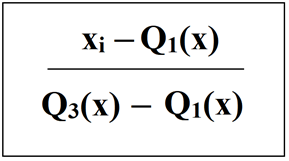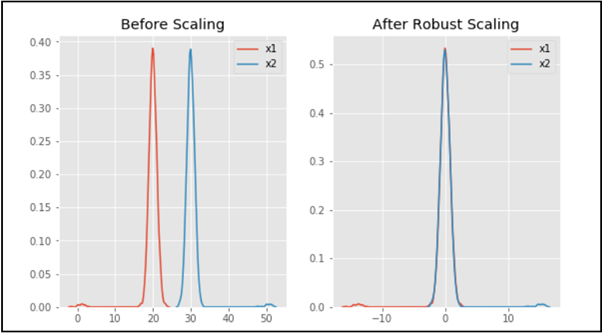Related Articles

# Feature Scaling – Part 3

• Last Updated : 26 Nov, 2020

Prerequisite – Feature Scaling | Set-1 , Set-2

Feature Scaling is one of the most important steps of Data Preprocessing. It is applied to independent variables or features of data. The data sometimes contains features with varying magnitudes and if we do not treat them, the algorithms only take in the magnitude of these features, neglecting the units. It helps to normalize the data in a particular range and sometimes also helps in speeding up the calculations in an algorithm.

Robust Scaler:

This uses a similar method to the Min-Max scaler but it instead uses the interquartile range, rather than the min-max, so that it is robust to outliers. This Scaler removes the median and scales the data according to the quantile range (defaults to IQR: Interquartile Range). The IQR is the range between the 1st quartile (25th quantile) and the 3rd quartile (75th quantile).
The formula below is used:Some more properties of Robust Scaler are:

• Robust Scaler as 0 mean and unit variance
• Robust Scaler has no predetermined range, unlike Min-Max Scaler
• Robust Scaler uses quartile ranges and this makes it less sensitive to outliers

Code:

 `# Python code for Feature Scaling using Robust Scaling`` ` `""" PART 1:  Importing Libraries """``import` `pandas as pd``import` `numpy as np``from` `sklearn ``import` `preprocessing``import` `matplotlib``import` `matplotlib.pyplot as plt``import` `seaborn as sns ``%` `matplotlib inline``matplotlib.style.use(``'ggplot'``)`` ` ` ` `""" PART 2:  Making the data distributions """``x ``=` `pd.DataFrame({``    ``# Distribution with lower outliers``    ``'x1'``: np.concatenate([np.random.normal(``20``, ``1``, ``2000``), np.random.normal(``1``, ``1``, ``20``)]),``    ``# Distribution with higher outliers``    ``'x2'``: np.concatenate([np.random.normal(``30``, ``1``, ``2000``), np.random.normal(``50``, ``1``, ``20``)]),``})`` ` ` ` `""" PART 3:  Scaling the Data """``scaler ``=` `preprocessing.RobustScaler()``robust_scaled_df ``=` `scaler.fit_transform(x)``robust_scaled_df ``=` `pd.DataFrame(robust_scaled_df, columns ``=``[``'x1'``, ``'x2'``])`` ` ` ` `""" PART 4:  Visualizing the impact of scaling """``fig, (ax1, ax2, ax3) ``=` `plt.subplots(ncols ``=` `3``, figsize ``=``(``9``, ``5``))``ax1.set_title(``'Before Scaling'``)``sns.kdeplot(x[``'x1'``], ax ``=` `ax1)``sns.kdeplot(x[``'x2'``], ax ``=` `ax1)``ax2.set_title(``'After Robust Scaling'``)``sns.kdeplot(robust_scaled_df[``'x1'``], ax ``=` `ax2)``sns.kdeplot(robust_scaled_df[``'x2'``], ax ``=` `ax2)`

Output:As you can see in the output, after Robust scaling, the distributions are brought into the same scale and overlap, but the outliers remain outside of bulk of the new distributions. Thus, Robust scaling is an effective method of scaling the data.

Attention reader! Don’t stop learning now. Get hold of all the important Machine Learning Concepts with the Machine Learning Foundation Course at a student-friendly price and become industry ready.

My Personal Notes arrow_drop_up